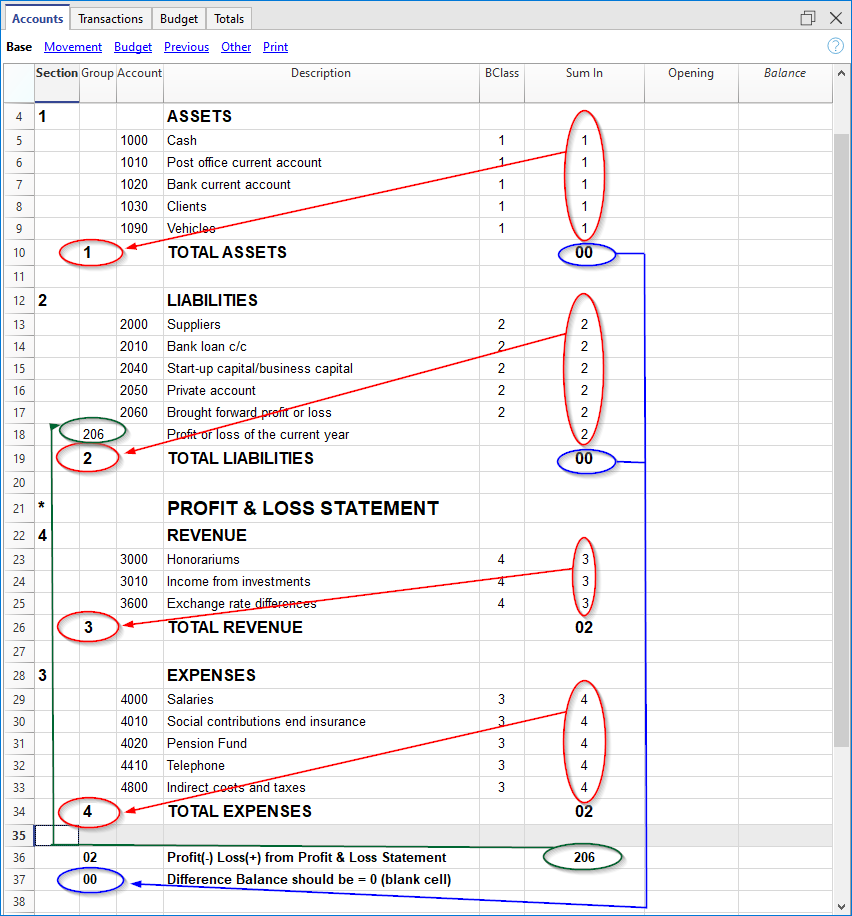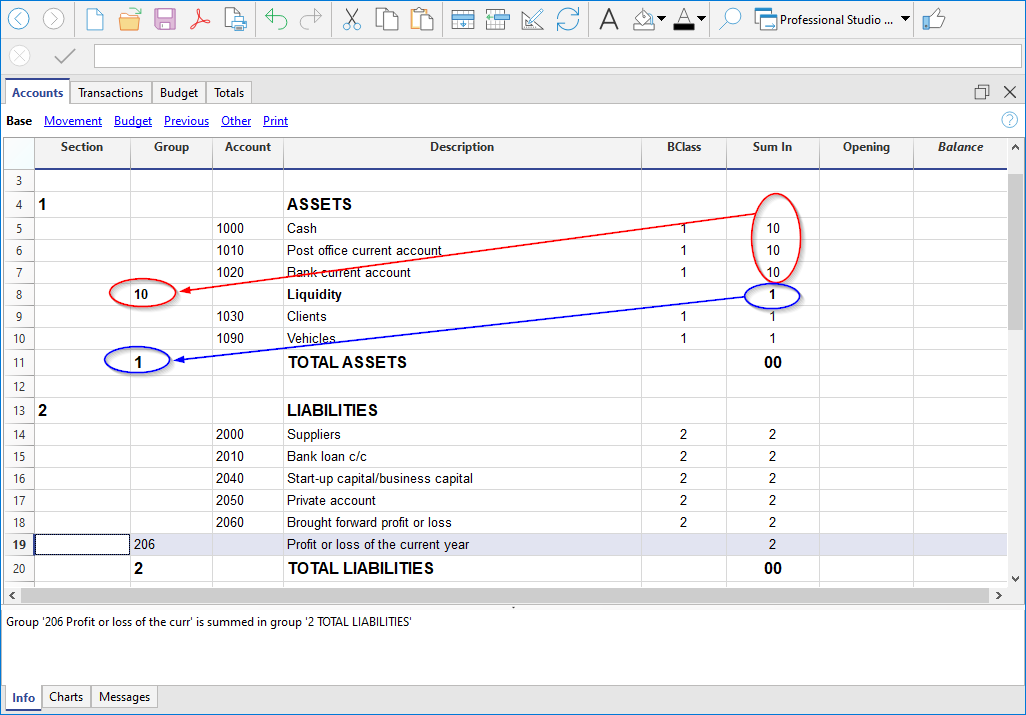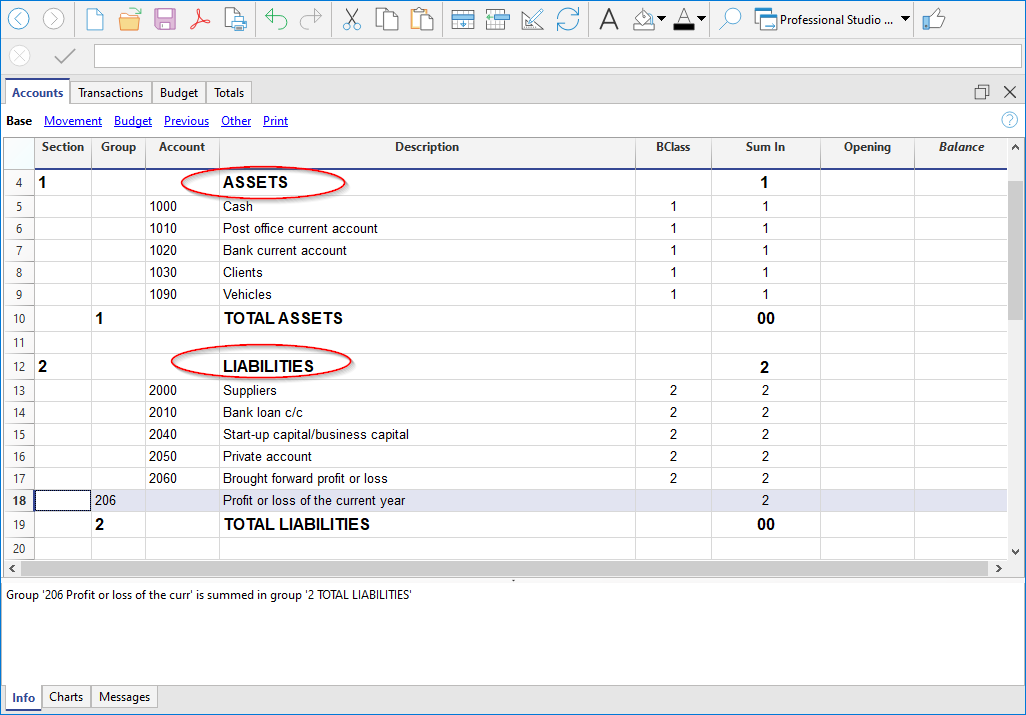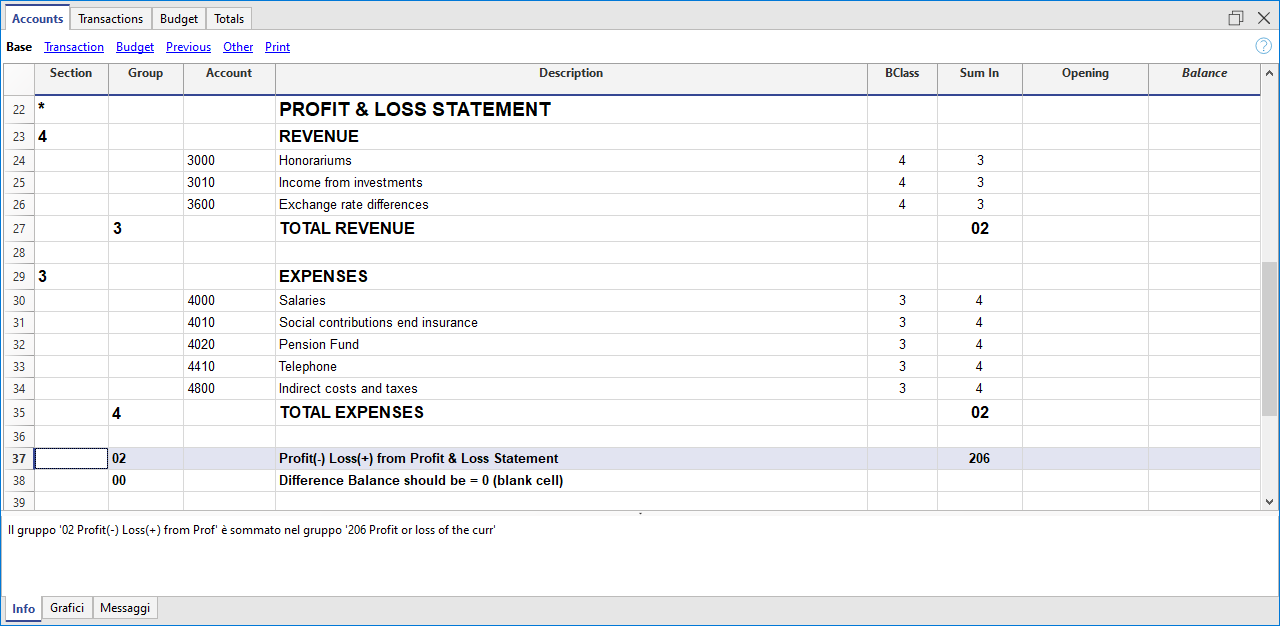# Groups

Documentation •

## The grouping and totaling system

Banana has developed a very practical and immediate grouping system, which allows you to set in the Accounts table all the information necessary to define the structure of the Balance sheet, Profit & loss statement and of other sections of the accounting.

The grouping system is flexible; it allows you to implement any national chart of accounts and at the same time to adapt it to the specific needs of your business. Both very simple and very complex plans and presentations can be created, with multiple levels of totalization, for each type of accounting.

Another advantage of this system is that it allows you to see the results immediately. Next to each account and group, you immediately see balance, movement and budget. Totals are updated instantly as you edit your file.

The Banana grouping and totaling system is based on two columns of the Accounts and Categories table:

• Group (Total row)
• When, in a row, a group identifier is being entered, the row becomes a TOTAL row.
• In this row the amounts of the Sum in column, that contain the same identifier, are being totaled.
• In a row, when a group is present, there cannot be an account.
• Sum in
• Sums the row amounts in the indicated group.
• For each account or group row, you indicate the group in which the line is to be totaled.
• The number here must be one of the numbers defined in the Group column.

## Set up of the Chart of Accounts structure

The chart of accounts in Banana Accounting can be set mainly in two different ways:

### 1. Structure without subgroups (or subtotals).

In this case, the structure is very simple. You list all accounts without subgroups, and total all accounts or categories in the main groups:

For Double-entry Accounting:

• Assets
• Liabilities
• Expenses
• Revenue

For Income/Expense Accounting or Cash Manager (Categories table):

• Income
• Expenses

### 2. Structure with subgroups

The structure is more complex. The accounts or categories of the main groups are divided into several subgroups, which in turn are totaled into the corresponding main groups.

Here we present a structure of the chart of accounts with an example of Subdivision with subgroups in Double-entry Accounting. Each subgroup can be in turn :

• #### Assets

• Current Assets
• Cash and Cash equivalents
• Customers
• Inventory
• Fixed Assets
• Furniture
• Machinery and equipment
• Computers, software.
• #### Liabilities

• Third-party capital
• Suppliers
• Bank loans
• Other short-term debts
• Long-term debts
• Equity
• Equity
• Reserve funds
• Profit and loss carried forward
• Profit for the year

From the Accounts table, at any time, through the menu File → Print or Print Preview you can print the chart of accounts or part of it.
Always starting from the Accounts table, by selecting the Transaction view, you have the printing of the verification balance.

## Examples of the main groups in the Double-entry accounting

Every accounting file template uses its own totaling system. Hereunder the main groups of the Double-entry accounting are being explained.

In the Double-entry accounting, the total of the Debit balances (positive) together with the Credit balances (negative) have to result in 0 (zero).

• The 00 is the control row where all the amounts are being added together.
• It is the "Grand total" of all the Debit & Credit balances.
• In case the Balances columns do not amount to zero, it means there is a Debit/Credit difference and thus most likely a mistake. In these cases it is useful to proceed with a checking of the accounting.
• In the Debit and Credit columns of the Transactions view (Accounts table), even if it is indicated that the Difference must be equal to 0, there are only positive amounts, so also in the row of the 00 group, there will be the total of the transactions not equal to 0.

The calculation sequence to arrive at 00 is therefore as follows:

• The 1000 accounts → group 1 (Total Assets) → Group 00
• The 2000 accounts → group 2 (Total Liabilities) → Group 00
• The 4000 accounts → group 4 (Total Expenses) → Group 02 (result Profit & Loss Statement)  →  Group 206 (Profit/Loss of the current year in the Balance Sheet) → Group 2 (Total Liabilities) → Group 00.
• The 3000 accounts → group 3 (Total Revenue) → Group 02 (result Profit & Loss Statement)  →  Group 206 (Profit/Loss of the current year in the Balance Sheet) → Group 2 (Total Liabilities) → Group 00.

### The result of profit & loss statement is added in equity

As you can see in the example, the Group 02 (Profit /Loss from Profit & Loss statement) is totalized in the 206 liabilities group (current year result).
With this group organization, we have several advantages:

• The current year operating result is displayed in the balance sheet
• The Total Liabilities will match the Total Assets (provided that there is no accounting error).• Position in the row preceding the one where the new group will be entered
• Type in the group column the group number, description, and the number of the column Sum in where you want this group to be totaled.

With this system it is easy to add totaling levels.
When we want to create a subgroup for the Cash & Cash equivalents accounts:

• Enter an empty row after the bank account
• Enter the value 10 into the Group column
• Enter the value 1 into the Sum in column
• Indicate the grouping 10 in columns Sum in the accounts 1000 and 1020
• The sequence for the calculation becomes:
The 1000 account → Group 10 (Cash & Cash equivalents) → Group 1 (Total Assets) → Group 00In case you want to insert another subgroup, "Current Assets", proceed in the same way.

• Add an empty row above the row of the Total Assets.
• In the new row:
• Indicate the number 11 in the Group column
• Indicate the number 1 in the Sum in column
• In the Clients and Goods for resale (inventory) rows, indicate the grouping 11 in column Sum in.

### Title rows (with Sum in)

Also in the title rows it is useful to indicate the grouping Sum in (the group of the total row) to which it belongs.
In this way, in the printouts of the Enhanced Balance Sheet by groups, if the accounts are zero, the title will not be printed.### Deleting subgroups

In case the Chart of accounts shows subgroups that are no longer needed or not wanted, these can be deleted. Just delete the row of the subgroup and modify the grouping Sum in of every account that was part of that subgroup.

### Checking of the structure

Once the Chart of accounts has been set up, execute the Actions menu → Check accounting command. In case there are errors, the program issues a warning.

### Infinite loop error

This warning appears when a Group is being totaled in a Group of a lower level, reason for which an infinite error loop is being created.
There would be an infinite loop when, in the preceding example, the Assets Group (1) would be totaled in Group 10.
The program, after having calculated the Group 1, would total the amount in Group 10, which in turn would total the amount in Group 1, and then again in 10 without ending.

## Profit & Loss Statement with Gross Profit

It is also possible to use a Profit & Loss Statement that starts with the total Business result and that subtracts the costs.
Hereunder the example of the Swiss PME Chart of Accounts is shown.## Extended use of the totaling system

The totaling system is very flexible:

• In one Group, it is possible to add Groups as well as Accounts
This can be seen in the earlier shown example where the Group Cash & cash equivalents (10) and the accounts (1030 e 1090) are being totaled in the Assets
• It is also possible to assign the group to the title rows
In this way, if in the printout the group is not printed because it is zero, the title is not printed either.
• You can use whatever kind of numbering (numeric and/or letters).
• You can create up to a hundred totaling levels
• The total is independent of the sequence:
• The Total row can be after the row
• The Total row can be before the rows
• The Total row can be completely elsewhere, in a separate position
This is being used to create registers (for example, a customer register)
• The accounts and the Groups can be totaled in one single other Group.
It is not possible to total one and the same account at the same time in two groups.
• This system is also being used to total the Cost centers and the Segments.
Pay attention though to not use different groups for normal accounts and for cost centers, because the resulting amounts will not be correct.

### Totaling of the Amount columns

The calculation procedure totals the amount columns

• The amount columns defined by the system are being totaled.
• The amount columns added by the user are being totaled.

### The sequence of the calculation

The program calculates the totals in the following manner:

• Sets the values of the Amount columns of the Groups rows on zero
• Totals the amounts of the Account rows into the Group rows (first calculation level)
• Adds the balance of the Group rows into the Group(s) of a superior level
Repeats the operation until all the levels have been calculated.